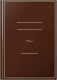Libros LibrosStraight lines which are parallel to the same straight line are parallel to one another. Triangles and Rectilinear Figures. The sum of the angles of a triangle is equal to two right angles.Elements of Geometry: Containing the First Six Books of Euclid with a ... - Página 29
por John Playfair - 1855 - 318 páginas
Vista completa - Acerca de este libro## Register

University of Cambridge - 1906
[ Lo sentimos, el contenido de esta página está restringido. ]
Vista de fragmentos - Acerca de este libro## The Church Missionary Juvenile Instructor

1854
...wonderful help that announcement used to be to us in mastering " Hie, hicc, hoc," ami proving that " straight lines which are parallel to the same straight line are parallel to one another !" Some seventy years ago there was a famous pirate named Captain Kidd. His plan was to give chase...
Vista completa - Acerca de este libro## A First Course of Geometry

...show that the straight lines which bisect the corresponding angles EFB, FGD are parallel. 35. PROP. 4. Straight lines which are parallel to the same straight line are parallel to one another. E /H -'K Let AB be parallel to LM and CD parallel to LM ; to prove that AB is parallel to CD. Draw...
Vista previa limitada - Acerca de este libro## Calendar

University of Birmingham - 1904
[ Lo sentimos, el contenido de esta página está restringido. ]
Sin vista previa disponible - Acerca de este libro## A complete text book of I.C.S.E. Mathematics

D.K. Bhattacharyya
[ Lo sentimos, el contenido de esta página está restringido. ]
Sin vista previa disponible - Acerca de este libro## A Shorter Geometry

....'.z.DGH + /.CGH=2rt. AS, II and, by (1), /.CGH = z.GHF, .'.LDGH +/.GHF = 2rt. Ls. q. KD THEOREM I. 6. Straight lines which are parallel to the same straight line are parallel to one another. fig. 272. Data AB, CD are each || to XY. To prove that AB is || to CD. Construction Draw a st. line...
Vista previa limitada - Acerca de este libro## The London University Calendar

London univ - 1874
...2. Divide a given rectilineal angle into two equal angles. 3. Define Alternate Angles; and show that straight lines which are parallel to the same straight line are parallel to one another. What fraction of a right angle is the exterior angle of a regular heptagon? 4. Show that all the interior...
Vista completa - Acerca de este libro## The New Geometry: Form One

G. P. West - 1965 - 316 páginas
...Since La = Lb, (proved in (i)) and since La + Ld=2 rt. LB, (ST stands on FSY) 1 = 2 rt. Ls. QED COR. Straight lines which are parallel to the same straight line are parallel to one another. ANGLE-SUM FOR TRIANGLE 237 THEOREM 6 (i). The sum of the angles of a triangle is equal to two right...
Vista previa limitada - Acerca de este libro## The New Zealand University Calendar, Volumen32

...are together equal to two right angles, then the two straight lines are parallel ; and the converse. Straight lines which are parallel to the same straight line are parallel to one another. Triangles and Rectilinear Figures. The sum of the angles of a triangle is equal to two right angles....
Vista completa - Acerca de este libro## Examinations Papers

1875
[ Lo sentimos, el contenido de esta página está restringido. ]
Vista de fragmentos - Acerca de este libro